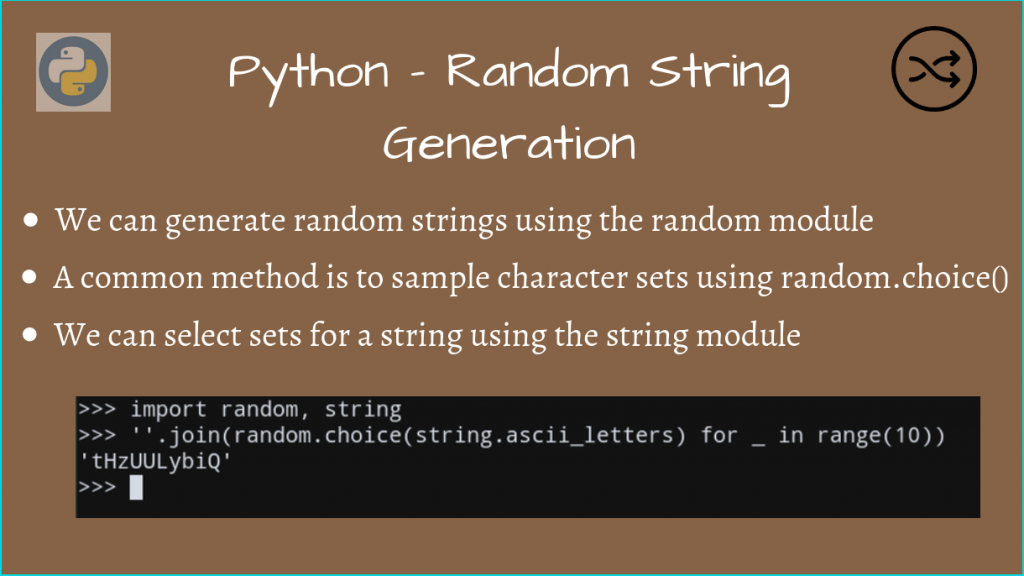# How to Generate Random Strings in PythonIn this article, we’ll take a look at how we can generate random strings in Python. As the name suggests, we need to generate a random sequence of characters, it is suitable for the `random` module.

There are various approaches here, so we’ll start from the most intuitive one; using randomized integers.

## Build a string from a random integer sequence

As you may know, the `chr(integer)` maps the integer to a character, assuming it lies within the ASCII limits. (Taken to be `255` for this article)

We can use this mapping to scale any integer to the ASCII character level, using `chr(x)`, where `x` is generated randomly.

```import random

# The limit for the extended ASCII Character set
MAX_LIMIT = 255

random_string = ''

for _ in range(10):
random_integer = random.randint(0, MAX_LIMIT)
# Keep appending random characters using chr(x)
random_string += (chr(random_integer))

print(random_string, len(random_string))

```

Sample Output

```ð|ÒR:
Rè 10
```

Here, while the length of the string seems to be 10 characters, we get some weird characters along with newlines, spaces, etc.

This is because we considered the entire ASCII Character set.

If we want to deal with only English alphabets, we can use their ASCII values.

```import random

random_string = ''

for _ in range(10):
# Considering only upper and lowercase letters
random_integer = random.randint(97, 97 + 26 - 1)
flip_bit = random.randint(0, 1)
# Convert to lowercase if the flip bit is on
random_integer = random_integer - 32 if flip_bit == 1 else random_integer
# Keep appending random characters using chr(x)
random_string += (chr(random_integer))

print(random_string, len(random_string))

```

Sample Output

```wxnhvYDuKm 10
```

As you can see, now we have only upper and lowercase letters.

But we can avoid all this hassle, and have Python do the work for us. Python has given us the `string` module for exactly this purpose!

Let’s look at how we can do the same this, using just a couple of lines of code!

## Generate Random Strings in Python using the string module

The list of characters used by Python strings is defined here, and we can pick among these groups of characters.

We’ll then use the `random.choice()` method to randomly choose characters, instead of using integers, as we did previously.

Let us define a function `random_string_generator()`, that does all this work for us. This will generate a random string, given the length of the string, and the set of allowed characters to sample from.

```import random
import string

def random_string_generator(str_size, allowed_chars):
return ''.join(random.choice(allowed_chars) for x in range(str_size))

chars = string.ascii_letters + string.punctuation
size = 12

print(chars)
print('Random String of length 12 =', random_string_generator(size, chars))
```

Here, we have specified the allowed list of characters as the `string.ascii_letters` (upper and lowercase letters), along with `string.punctuation` (all punctuation marks).

Now, our main function was only 2 lines, and we could randomly choose a character, using `random.choice(set)`.

Sample Output

```abcdefghijklmnopqrstuvwxyzABCDEFGHIJKLMNOPQRSTUVWXYZ!"#\$%&'()*+,-./:;<=>?@[\]^_`{|}~
Random String of length 12 = d[\$Om{;#cjue
```

We have indeed generated a random string, and the `string` module allows easy manipulations between character sets!

### Make the random generation more secure

While the above random generation method works, if you want to make your function be more cryptographically secure, use the `random.SystemRandom()` function.

An example random generator function is shown below:

```import random
import string

output_string = ''.join(random.SystemRandom().choice(string.ascii_letters + string.digits) for _ in range(10))

print(output_string)
```

Output

```iNsuInwmS8
```

This ensures that your string generation is cryptographically secure.

## Random UUID Generation

If you want to generate a random UUID String, the `uuid` module is helpful for this purpose.

```import uuid

# Generate a random UUID
print('Generated a random UUID from uuid1():', uuid.uuid1())
print('Generated a random UUID from uuid4():', uuid.uuid4())
```

Sample Output

```Generated a random UUID from uuid1(): af5d2f80-6470-11ea-b6cd-a73d7e4e7bfe
Generated a random UUID from uuid4(): 5d365f9b-14c1-49e7-ad64-328b61c0d8a7
```

## Conclusion

In this article, we learned how we could generate random strings in Python, with the help of the `random` and `string` modules.